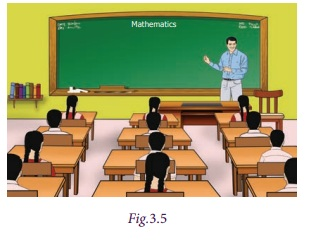Home | | Maths 7th Std | Value of an algebraic expression

# Value of an algebraic expression

Sometimes we would like to assign definite values to the variables in an expression to find the value of that expression. This situation arises in many real life problems.

Value of an algebraic expression

Sometimes we would like to assign definite values to the variables in an expression to find the value of that expression. This situation arises in many real life problems.

For example, if the teacher of class 7 wants to select 10 students for a competition, for which she can choose any number of boys and girls.If the number of boys are x and the number of girls are y, then the required algebraic expression to find the total number of participants is x + y.

Here, the total number of students to be selected is 10 and if only two girls are interested to participate in the competition, then how many boys should be selected? Obviously, if y = 2 then x + 2 = 10. The value x = 8 satisfies the equation. So, the number of boys to be selected is 8.

Follow the steps to obtain the value.

Step − 1: Study the problem. Fix the variable and write the algebraic expression.

Step − 2: Replace each variable by the given numerical value to obtain an arithmetical expression.

Step − 3: Simplify the arithmetical expression by BIDMAS method.

Step − 4: The value so obtained is the required value of the expression.

Try this

Try to find the value of the following expressions, if p = 5 and q = 6.

i) p + q ii) qp iii) 2p + 3q iv) pqpq v) 5pq – 1

i) p + q = 5 + 6 = 11

ii) q – p = 6 – 5 = 1

(iii) 2p + 3q = 2(5)+ 3(6)

10 + 18 = 28

iv) pq – p – q = 5 × 6 –5 – 6

30 – 11 = 19

v) 5pq – l = 5 × 5 × 6 – 1

150 – 1 = 149

Example 3.3

If x = 3, y = 2 find the value of (i) 4x + 7y (ii) 3x + 2y − 5 (iii) xy

Solution

(i) 4x + 7y = 4 (3) + 7 (2) = 12 + 14 = 26

(ii) 3x + 2y – 5 = 3 (3) + 2 (2) – 5 = 9 + 4 – 5 = 8

(iii) x y = 3 – 2 = 1

Example 3.4

Find the value of (i) 3m + 2n (ii) 2mn (iii) mn − 1, given that m = 2, n = − 1.

Solution

(i) 3m + 2n = 3(2) + 2( −1) = 6 −2 = 4

(ii) 2mn = 2(2) − ( −1) = 4 + 1 = 5

(iii) mn −1 = (2) ( −1) −1 = −2 −1 = –3

Tags : Algebra | Term 1 Chapter 3 | 7th Maths , 7th Maths : Term 1 Unit 3 : Algebra
Study Material, Lecturing Notes, Assignment, Reference, Wiki description explanation, brief detail
7th Maths : Term 1 Unit 3 : Algebra : Value of an algebraic expression | Algebra | Term 1 Chapter 3 | 7th Maths